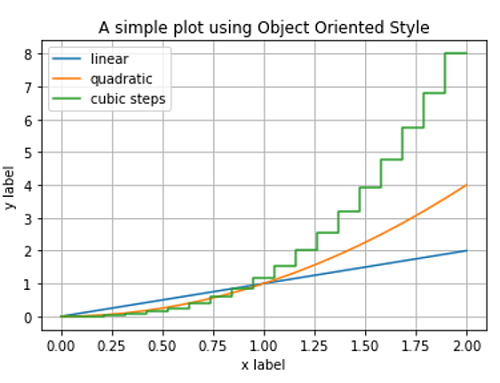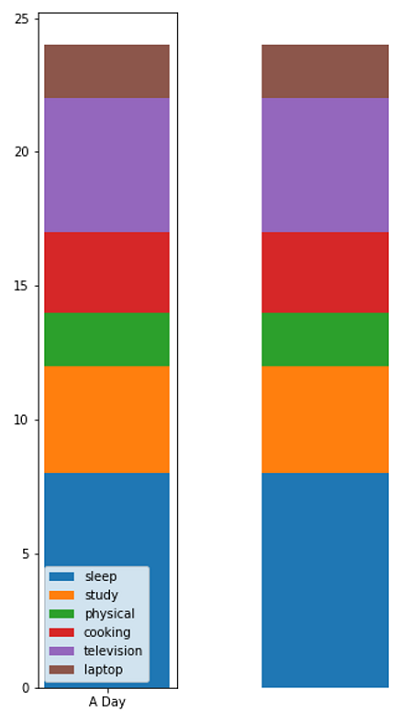# Python | Object Oriented Style Plotting in Matplotlib

In this tutorial, we are going to learn how to plot figures using an object-oriented style in matplotlib?
Submitted by Anuj Singh, on August 17, 2020

There are two ways to do plotting in Matplotlib,

• Creating axes and figures explicitly by initializing objects for a figure. We can call methods on them and therefore it is called as object-oriented (OO) style.
• Pyplot automatically creates and manages the figures and axes. We can use matplotlib.pyplot inbuilt defined functions for plotting and pyplot api itself calculates to operate on figures.

Illustrations:## Python for code object-oriented style plotting in matplotlib

```import numpy as np
import matplotlib.pyplot as plt

x = np.linspace(0, 2, 20)

fig, ax = plt.subplots()
ax.plot(x, x, label='linear')
ax.step(x, x**3, label='cubic steps')
ax.set_xlabel('x label')
ax.set_ylabel('y label')
ax.set_title("A simple plot using Object Oriented Style")
ax.legend()
ax.grid()

plt.figure()
plt.plot(x, x, label='linear')
plt.step(x, x**3, label='cubic steps')
plt.xlabel('x label')
plt.ylabel('y label')
plt.title("A simple plot using automatically created by pyplot")
plt.legend()
plt.grid()
```

Output:

```Output is as Figure
```

Languages: » C » C++ » C++ STL » Java » Data Structure » C#.Net » Android » Kotlin » SQL
Web Technologies: » PHP » Python » JavaScript » CSS » Ajax » Node.js » Web programming/HTML
Solved programs: » C » C++ » DS » Java » C#
Aptitude que. & ans.: » C » C++ » Java » DBMS
Interview que. & ans.: » C » Embedded C » Java » SEO » HR
CS Subjects: » CS Basics » O.S. » Networks » DBMS » Embedded Systems » Cloud Computing
» Machine learning » CS Organizations » Linux » DOS
More: » Articles » Puzzles » News/Updates